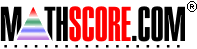Math Practice Online > free > lessons > Florida > 6th grade > Probability

## Probability

This topic covers probability problems. You may need pencil and paper.

 Sample Problems for Probability Lesson for Probability

### This topic aligns to the following state standards

Grade 3: Dat 3. Calculates the probability of a particular event occurring from a set of all possible outcomes.
Grade 4: Dat 3. Calculates the probability of a particular event occurring from a set of all possible outcomes.
Grade 5: Dat 3. Calculates the probability of a particular event occurring from a set of all possible outcomes.
Grade 6: Dat 2. Calculates simple mathematical probabilities.
Grade 7: Dat 3. Calculates simple mathematical probabilities for independent and dependent events.
Grade 8: Dat 2. Calculates simple mathematical probabilities for independent and dependent events.
Grade 9: Dat 2. determines the probability for simple and compound events as well as independent and dependent events
Grade 4: Dat 1. Identifies and records using common fractions, the possible outcomes of simple experiments using concrete materials (for example, spinners, number cubes, coin toss).
Grade 4: Dat 2. Determines and predicts which outcomes are likely to occur and expresses those expected outcomes as fractions.
Grade 5: Dat 2. Explains and predicts which outcomes are most likely to occur and expresses the probabilities as fractions.
Grade 6: Dat 1. Examines and describes situations that include finding the odds for and against a specified outcome.
Grade 7: Dat 1. Computes the mathematical odds for and against a specified outcome in given real-world experiments.
Grade 8: Dat 1. Predicts the mathematical odds for and against a specified outcome in a given real-world situation.

Copyright Accurate Learning Systems Corporation 2008.